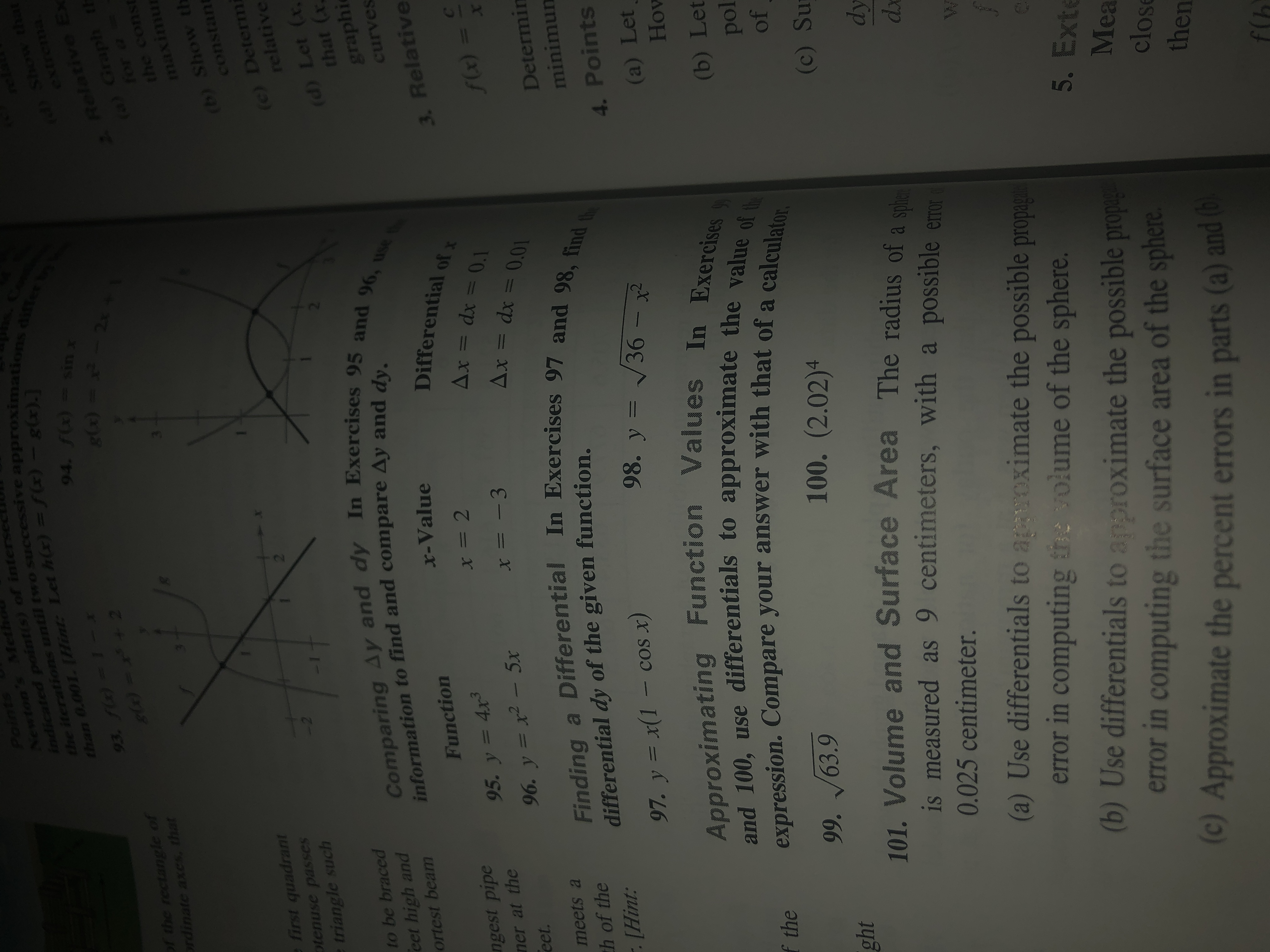# PoinNewton's Metindicated poimtis) of intersethe iterations until two successive approximationsthan 0.001.Hint: Let hx) = f) - g(x).]94. f(x)= sinxgx) = _ 2) Show that93. fl)=1-admxoof the rectangle ofrdinate axes, thatRelative Ex(a) Graph thfor a =the constmaximu(b) Show thconstantefirst quadranttenuse passestriangle such2(c) Determrelative2Comparing Ay and dy In Exercises 95 and 96, useto be bracedeet high andortest beam(d) Let (xthat (xgraphicurvesinformation to find and compare Ay and dy.X-ValueDifferential ofxFunctionngest pipener at theeet.3. Relative= 295. y= 4xAx = dx = 0.1=- 396. y = x- 5xAx = dx = 0.01f(x) =XFinding a DifferentialIn Exercises 97 and 98, findmeets ath of the. [Hint:Determindifferential dy of the given function.minimun97. y =x(1 - cos x)98. y = 36 -4. PointsApproximating Function Values In Exercisesand 100, use differentials to approximate the value of theexpression. Compare your answer with that of a calculator.(a) LetHow(b) Letpoloff the99.63.9100. (2.02)4101. Volume and Surface Area The radius of a speis measured as 9 centimeters, with a possible emoght(c) Sudy0.025 centimeter.dx(a) Use differentials to agaroximate the possible propagherror in computing the volume of the sphere.(b) Use differentials to approximate the possible propaerror in computing the surface area of the sphere(c) Approximate the percent errors in parts (a) and to5. ExtMeaclosethenf(b6

Question
6 views

97help_outlineImage TranscriptionclosePoin Newton's Met indicated poimtis) of interse the iterations until two successive approximations than 0.001.Hint: Let hx) = f) - g(x).] 94. f(x)= sinx gx) = _ 2 ) Show that 93. fl)=1- admxo of the rectangle of rdinate axes, that Relative Ex (a) Graph th for a = the const maximu (b) Show th constant efirst quadrant tenuse passes triangle such 2 (c) Determ relative 2 Comparing Ay and dy In Exercises 95 and 96, use to be braced eet high and ortest beam (d) Let (x that (x graphi curves information to find and compare Ay and dy. X-Value Differential ofx Function ngest pipe ner at the eet. 3. Relative = 2 95. y= 4x Ax = dx = 0.1 = - 3 96. y = x- 5x Ax = dx = 0.01 f(x) = X Finding a DifferentialIn Exercises 97 and 98, find meets a th of the . [Hint: Determin differential dy of the given function. minimun 97. y =x(1 - cos x) 98. y = 36 - 4. Points Approximating Function Values In Exercises and 100, use differentials to approximate the value of the expression. Compare your answer with that of a calculator. (a) Let How (b) Let pol of f the 99.63.9 100. (2.02)4 101. Volume and Surface Area The radius of a spe is measured as 9 centimeters, with a possible emo ght (c) Su dy 0.025 centimeter. dx (a) Use differentials to agaroximate the possible propagh error in computing the volume of the sphere. (b) Use differentials to approximate the possible propa error in computing the surface area of the sphere (c) Approximate the percent errors in parts (a) and to 5. Ext Mea close then f(b 6 fullscreen
check_circle

Step 1

The given equa...

### Want to see the full answer?

See Solution

#### Want to see this answer and more?

Solutions are written by subject experts who are available 24/7. Questions are typically answered within 1 hour.*

See Solution
*Response times may vary by subject and question.
Tagged in

### Calculus